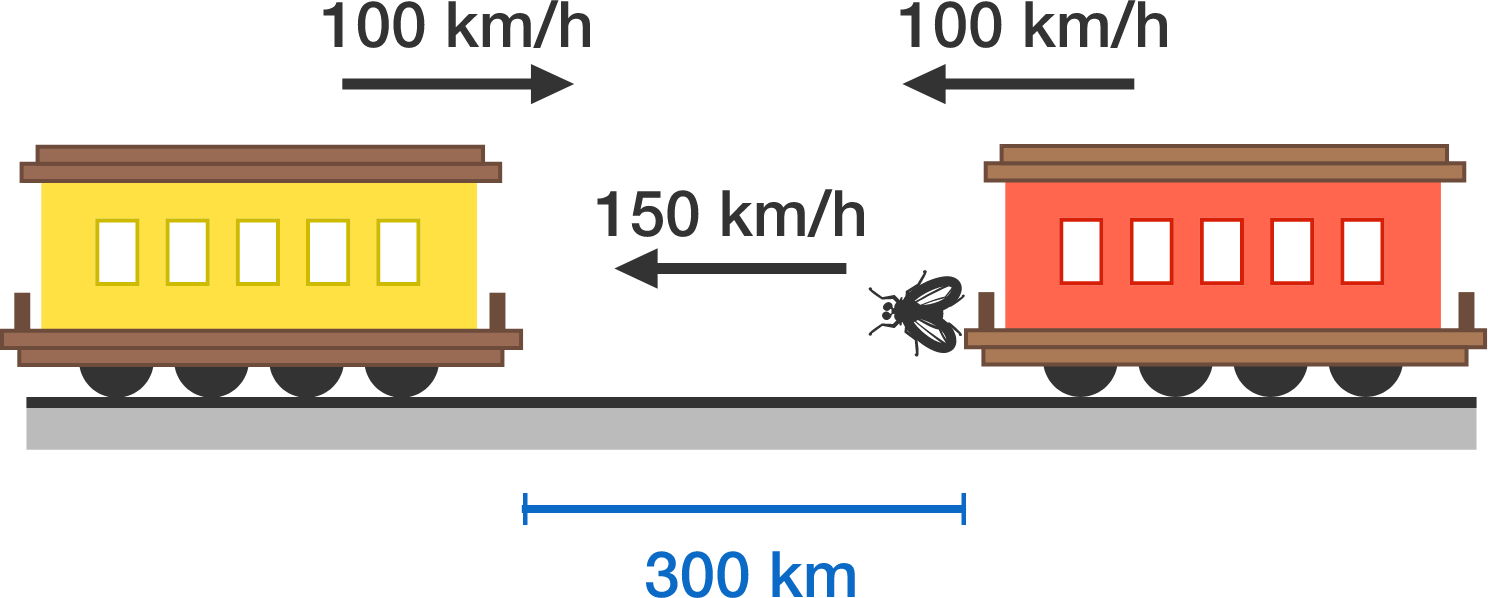# 76 of 100: Daredevil Fly

Algebra Level 1Two trains, each traveling at a speed of $\SI[per-mode=symbol]{100}{\kilo\meter\per\hour},$ are headed towards each other on a straight track. At the exact moment the trains are $\SI{300}{km}$ apart, a super-fast fly on the front of one train begins flying back and forth between the two trains at a speed of $\SI{150}{km/h}.$

What is the total distance the fly will have traveled before getting crushed between the trains?There is a very simple way to calculate how far the fly flies, so think carefully!

×